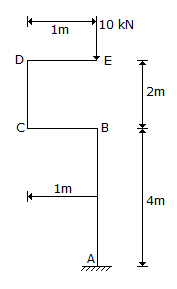# Civil Engineering - UPSC Civil Service Exam Questions

16.

Which one of the following equations correctly gives the relationship between the specific graivity of soil grains (G) and the hydraulic gradient (i) to initiate 'quick' condition in a sand having a void ratio of 0.5 ?

 A. G = 0.5 i + 1 B. G = i + 0.5 C. G = 1.5 i + 1 D. G = 1.5 i - 1

Explanation:

No answer description available for this question. Let us discuss.

17.

What is the bending moment at A for the bent column shown in the D figure ?A. 40 kNm B. 20 kNm C. 10 kNm D. Zero C

Explanation:

No answer description available for this question. Let us discuss.

18.

Consider the following statements :
The principle of superpositions is applied to :
1. Linear elastic bodies
2. Bodies subjected to small deformations.
Of these statements :

 A. 1 alone is corrrect B. 1 and 2 are correct C. 2 alone is correct D. neither 1 nor 2 is correct

Explanation:

No answer description available for this question. Let us discuss.

19.

In which type of lakes, does a perfect ecological equilibrium among the producers, decomposers and consumer groups of organisms exist ?

 A. Senescent lakes B. Mesotrophic lakes C. Oligotrophic lakes D. Eutrophic lakes

Explanation:

No answer description available for this question. Let us discuss.

20.

A soil deposit has three layers having same thickness each but the permeabilities of the layers are in the ratio of 1 : 2 : 4 from top to bottom. What is the ratio of average permeability in the horizontal direction to that in the vertical direction ?

 A. 7 : 2 B. 14 : 6 C. 28 : 24 D. 49 : 36